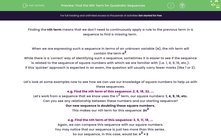# Find the Nth Term for Quadratic Sequences

In this worksheet, students will apply knowledge of square numbers to find the nth term for quadratic sequences and apply these rules to find unknown terms.Key stage:  KS 4

Year:  GCSE

GCSE Subjects:   Maths

GCSE Boards:   AQA, Eduqas, Pearson Edexcel, OCR,

Curriculum topic:   Algebra

Curriculum subtopic:   Sequences

Popular topics:   Square Numbers worksheets

Difficulty level:#### Worksheet Overview

Finding the nth term means that we don't need to continuously apply a rule to the previous term in a sequence to find a missing term.

When we are expressing such a sequence in terms of an unknown variable (n), the nth term will contain the term n2.

While there is a 'correct' way of identifying such a sequence, sometimes it is easier to see if the sequence is related to the sequence of square numbers with which we are familiar with (i.e. 1, 4, 9, 16, etc.).

If this 'quicker' approach is expected in an exam, the question will usually carry fewer marks (like 1 or 2).

Let's look at some examples now to see how we can use our knowledge of square numbers to help us with these sequences.

e.g. Find the nth term of this sequence: 2, 8, 18, 32, ...

Let's work from a sequence that we know uses the n2 term, our square numbers: 1, 4, 9, 16, etc.

Can you see any relationship between these numbers and our starting sequence?

Our new sequence is doubling these square numbers.

This makes our nth term for this sequence: 2n2

e.g. Find the nth term of this sequence: 3, 6, 11, 18, ...

Again, we can compare this sequence with our square numbers.

You may notice that our sequence is just two more than this series.

So our sequence, in this case, would be: n2 + 2

e.g. Find the nth term of this sequence: 19, 16, 11, 4, ...

If we compare this to our square numbers, you may notice that they add up to 20.

This could be looked at like this: "If we take the square numbers away from 20, we reach our target."

This makes our nth term in this case: 20 - n2

In this activity, we will apply our knowledge of square numbers to find the nth term for quadratic sequences and apply these rules to find unknown terms.

If you are not feeling totally confident with your square numbers, you may want to revise these before you attempt this activity.

### What is EdPlace?

We're your National Curriculum aligned online education content provider helping each child succeed in English, maths and science from year 1 to GCSE. With an EdPlace account you’ll be able to track and measure progress, helping each child achieve their best. We build confidence and attainment by personalising each child’s learning at a level that suits them.

Get started